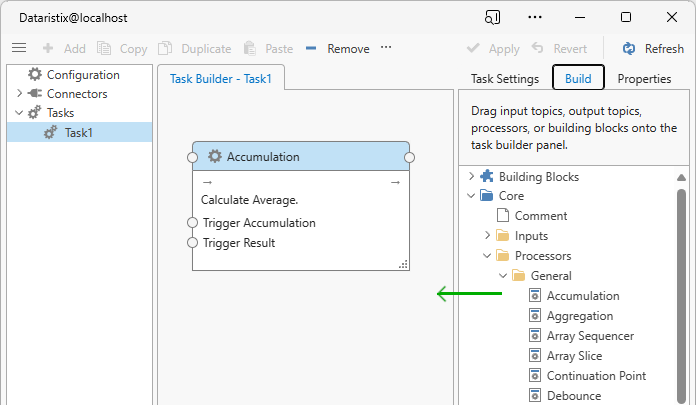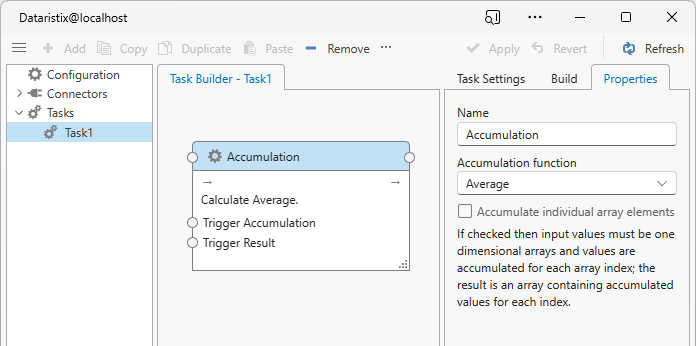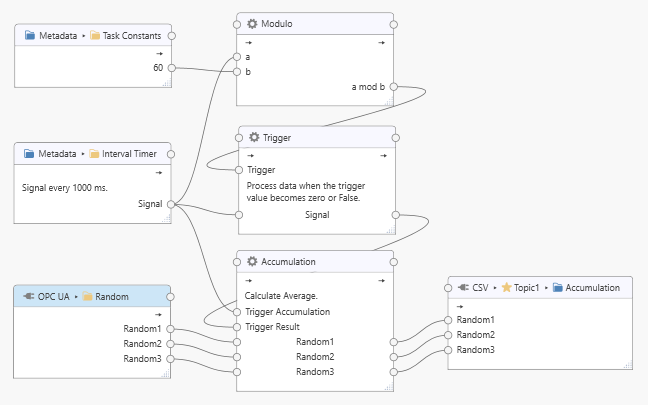# Accumulation Processor¶

The “Accumulation Processor” is installed with the Dataristix Application. The processor accumulates each connected input value over time using a selected function.

If an input value is a one-dimensional array then the accumulated value is calculated over all array elements of the input value over time.

If an input value is of “uncertain” quality then the output value will also be of uncertain quality.

If an input value is of “bad” quality then the output value will also be of bad quality.## Configuration¶

Select the “Accumulation Processor” item in the “Task Builder” panel to view its properties.You can change the following properties:

• Topic
The title of the “Accumulation Processor” as it appears within the task builder.
• Accumulation Function
• Average
• Count (number of samples)
• Minimum
• Maximum
• Standard Deviation (Population)
• Standard Deviation (Samples)
• Sum

Press the “Apply” button to commit your changes.

## Input Tags¶

The processor has two trigger inputs and you can connect additional value inputs for accumulation by dragging connection points onto the topic connection point.

The trigger inputs perform the following task:

• Trigger Accumulation
Whenever the value for the Trigger Accumulation input changes then all current value inputs are accumulated using the selected function. Note that the standard deviation functions require additional memory to store interim values during the accumulation period.
• Trigger Result
Whenever the value for the Trigger Result input changes then the accumulation result for each value input is passed on to the next stage within the task and the accumulation is reset.

## Example¶

The following example shows a task that calculates the average of OPC UA value inputs “Random1”, “Random2” and “Random3” over one minute while accumulating values every second before storing the accumulated data into a file.The Interval Timer is used to send a one-second signal as an incrementing counter. The Signal value is connected to the Trigger Accumulation input of the Accumulation processor to accumulate values every second.

A 60 second interval is derived from the same timer by using the Modulo math processor. When the modulo value reaches zero after one minute then the connected Trigger processor passes the Signal value onto the Trigger Result input of the Accumulation processor. Since the Signal value always changes, it will trigger the accumulation result to be calculated and passed onto the CSV processor for storage.